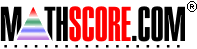Math Practice Online > free > lessons > Florida > 6th grade > Decimal Subtraction

## Decimal Subtraction

Subtraction with decimals. You may need pencil and paper.

 Sample Problems for Decimal Subtraction Lesson for Decimal Subtraction

### This topic aligns to the following state standards

Grade 5: Num 1. Uses problem-solving strategies to determine the operation(s) needed to solve one- and two- step problems involving addition, subtraction, multiplication, and division of whole numbers, and addition, subtraction, and multiplication of decimals and fractions.
Grade 6: Num 1. Knows the effects of the four basic operations on whole numbers, fractions, mixed numbers, and decimals.
Grade 7: Num 1. Knows the effects of the four basic operations on whole numbers, fractions, mixed numbers, and decimals.
Grade 8: Num 1. Knows the effects of the four basic operations on whole numbers, fractions, mixed numbers, decimals, and integers.

Copyright Accurate Learning Systems Corporation 2008.